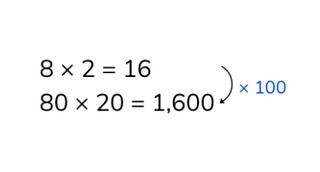Multiplying with tens

# Multiplying with tens

Students learn to multiply with tens numbers.

No account needed.8,000 schools use Gynzy92,000 teachers use Gynzy1,600,000 students use Gynzy

## General

Students learn to multiply with two tens numbers.

## Standards

CCSS.Math.Content.4.NBT.A.1

## Learning objective

Students will be able to multiply with two multiples of ten.

## Introduction

Have the students calculate as many multiplication problems as they can in the time given.

## Instruction

How the problem on the interactive whiteboard and ask how the students would solve it. Next, show that you can solve the problem in steps by first crossing out the zeros and multiplying the remaining numbers. Then you put the correct number of zeros back at the end of the answer. Explain that you can first solve the problem without zeros. This is how you calculate the answer in steps if you add the zeros back on. The answer is thus 100 times as much in the example. Have the students solve a few problems and ask how they did this. Finally, show how you can solve a story problem. You have the students determine what kind of problem it is, which numbers you nee to make the problem, and solve it. Then have the students solve the other story problems on their own.

Check whether the students can multiply with two tens numbers with the following question:
- How do you solve the problem 80 × 40?

## Quiz

Students test their understanding of multiplying with tens numbers in ten exercises. For some of these they have possible answers to choose from, others they must provide the answer on their own, and others are in the form of a story problem.

## Closing

You discuss once again with the students that it is important to be able to multiply with two tens numbers, because that is how you can solve multiplication problems with large numbers. As a closing activity you divide the class up into an even number of groups. Each group competes against another group. The entire class calculates the problem that you state. Between the two teams that are competing, the one that has the correct answer first gets a point. The groups keep count of their own points. State several more problems. Which group has the most points at the end?

## Teaching tips

Have students that have difficulty with multiplying with two tens numbers first practice with multiplication problems for which there is only one tens number. Then they practice problems with two tens numbers.

### The online teaching platform for interactive whiteboards and displays in schools

• Save time building lessons

• Manage the classroom more efficiently

• Increase student engagement